# From Simple to Chaotic Pendulum Systems in Wolfram|Alpha

March 3, 2011 —Comments Off

Students of the history of science will recall learning that Galileo observed and described the periodic motion of a simple pendulum around 1602. Until being supplanted by other technologies around the first third of the 20th century, this property of pendula has been indispensible in the creation of accurate timekeeping devices.

An idealized pendulum consisting of a weight (often called a “bob”) on the end of a massless cord and suspended from a frictionless pivot is called a simple pendulum or, more explicitly, a simple gravity pendulum. Wolfram|Alpha has known about simple pendula for some time, as you can verify by entering “pendulum”. In fact, doing so brings up not one but two pendulum results:

As you can see, Wolfram|Alpha returns both the usual (small-amplitude approximation) pendulum as well as the full pendulum (correct for arbitrary swing angles). The screenshot above shows the oscillation period calculated for the first of these cases, using some default values for the variables; comparing the resulting oscillation period with that of the full pendulum (further down the result) shows a difference—albeit a very small one—as one might expect. Since the formulas here are simple, clicking “Show formula” in each of the two subpods displays the actual formula describing the period, where the function K(m) in the second case denotes the so-called complete elliptic integral of the first kind: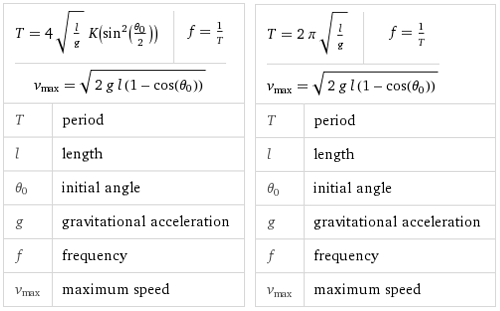Some additional and useful graphical information is also shown in each case. In particular, the phase portraits show that the behavior described by the equations of motion is similar in both cases for small angles ?, but differs substantially for larger angles (the small-amplitude case corresponding to simple harmonic motion and the general case to the actual pendulum, which in particular can exhibit circulation in addition to oscillation):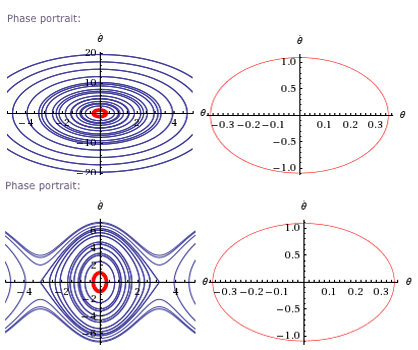But that’s old news. The new news is that Wolfram|Alpha now knows about more complicated (and therefore more interesting!) systems, including multiple and linked pendula. For example, if two pendula are linked together, they form a double pendulum: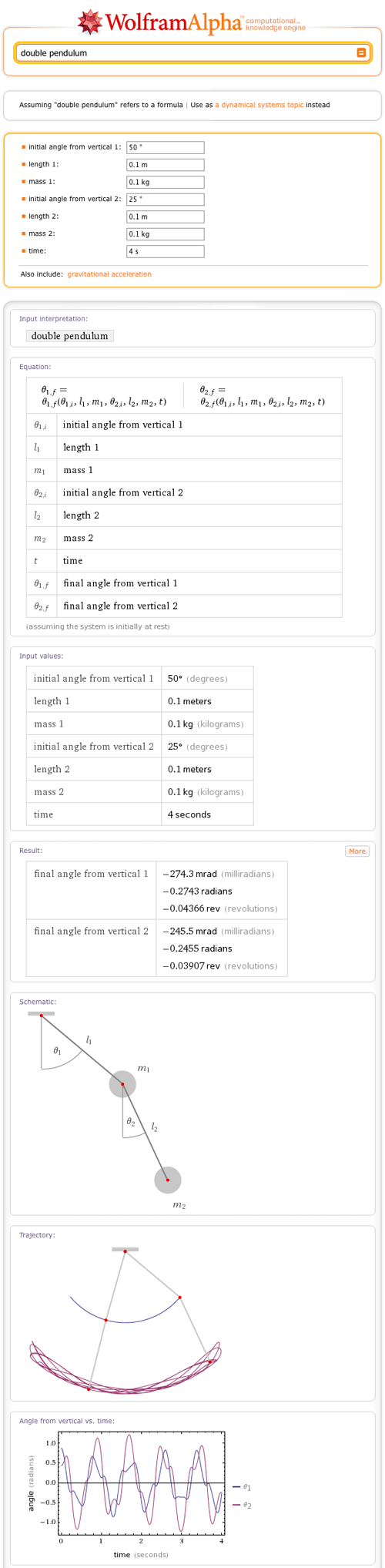The motion of the double pendulum is completely described by its (complicated) equations of motion, but its behavior depends sensitively to small changes in initial conditions. This is one of the hallmarks of chaos, and, in fact, the motion of the double pendulum can be chaotic. You can explore this sensitivity on your own by changing some initial values to see how dramatically the motion changes—for example, with the input “double pendulum, theta2=-21deg”: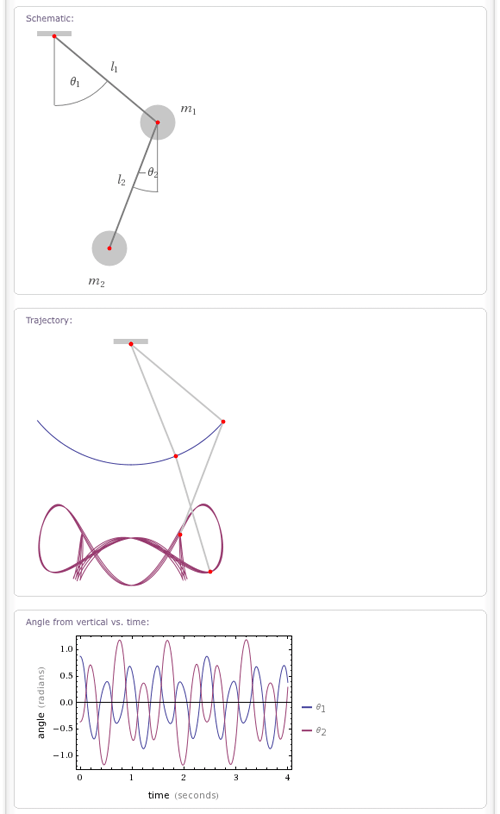Another example is the spring pendulum, in which the string is replaced by a spring. While in a simple pendulum, the length of the string is constant and so is the period, for a spring penfulum, the length of a spring can also change. This additional degree of freedom gives rise to chaotic behavior: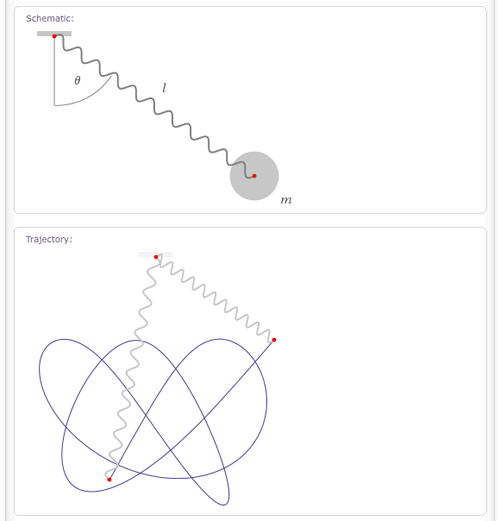Similarly, you can now explore the behavior of coupled pendula in Wolfram|Alpha: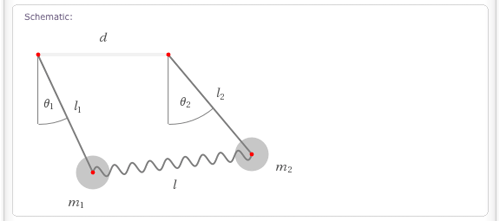The results returned for most of these systems also display useful physical properties, such as the Lagrangian and equations of motion—here, for example, for the double pendulum: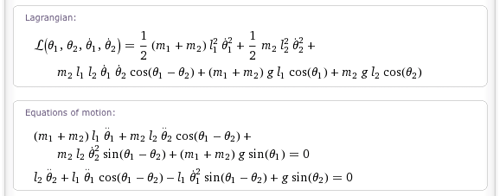There is clearly much more work that can be done in this direction, and among the features being worked on are animations, phase portraits, and an entire catalog of named physical systems and their properties. So some day in the not-so-distant future, you should be able to ask Wolfram|Alpha for the “Euler-Lagrange equations of motion of a heavy symmetric top” and get not only a result, but also computable data in terms of NDSolve solutions. Stay tuned!

This post was written by Eric Weisstein and Toni Schindler.